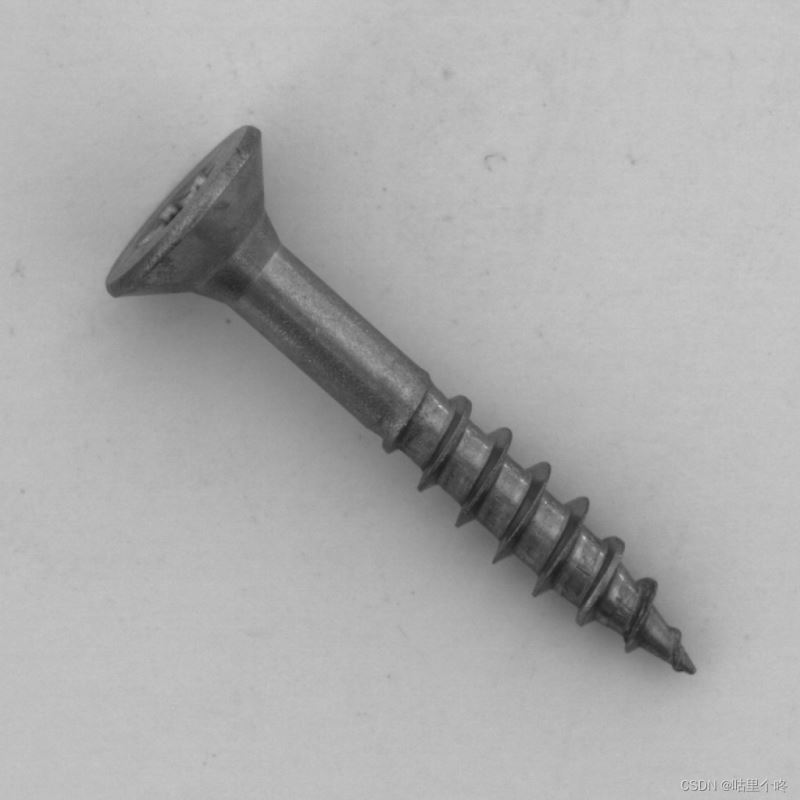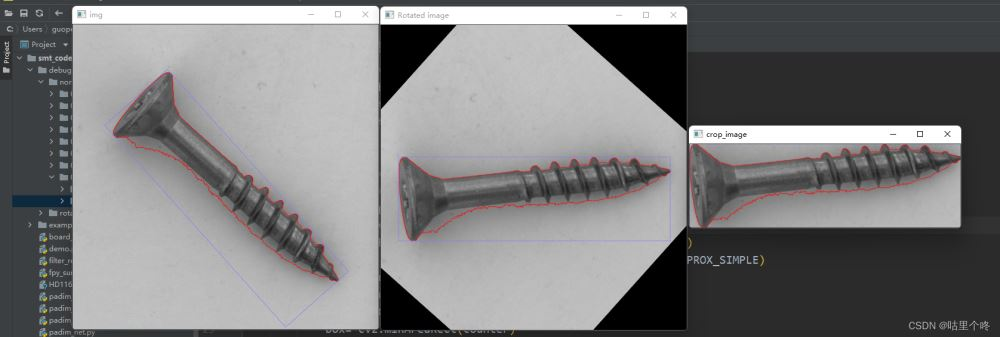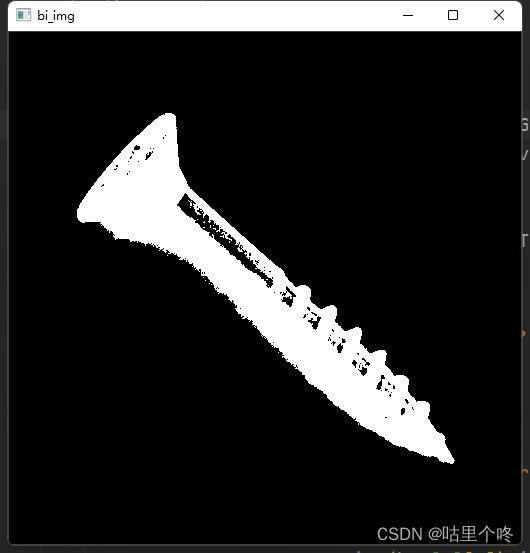﻿ Opencv实现倾斜图片转正示例_python_脚本之家
python# Opencv实现倾斜图片转正示例## 实现步骤：

1.灰度化+二值化,效果图如下2.找轮廓，cv2.findcounters()，这样的图我们肯定会找到很多轮廓，所以我们可以通过面积来筛选出自己想要的那个轮廓，这里我们选择最大的轮廓，也就是钉子的轮廓，看看效果（红线就是哦我们找到的轮廓点然后连接起来的）3.找这个轮廓的最小外接矩形（带角度），这个就是最关键的了，也就是我们开头提到的cv2.minAreaRect()，我们要对他的返回值了如执掌。先看看效果呢（蓝线就是我们找到的最小外接矩形）这个时候你会说，这有什么难的，我们来细细看一下，cv2.minAreaRect()的返回值是什么

```box= cv2.minAreaRect(counter)
print(box)```

`((257.3854675292969, 292.03851318359375), (454.5963439941406, 140.96072387695312), 48.21548080444336)`

`((290.1945495605469, 256.9798889160156), (140.9435577392578, 460.39862060546875), 49.1729850769043)`我想我已经说明白了

4.根据得到的中心点和角度以及第二个元组的值的大小就可以开始旋转了5.有中心点和两条边长的大小，我们就可以开始抠图了，看看效果```# -*- coding: utf-8 -*-
# @Time : 2022/7/25 15:51
# @Author : guligedong
import cv2
import os
import numpy as np

base_folder = r'F:\mvtec_anomaly_detection\screw\test\good'
for i in os.listdir(base_folder):
img_path = os.path.join(base_folder, i)
if img_path.split('.')[-1] == 'db':
continue
print(img_path)
img = cv2.resize(img,(512,512))
img_h,img_w = img.shape[:2]
gray_img = cv2.cvtColor(img, cv2.COLOR_BGR2GRAY)
_,bi_img = cv2.threshold(gray_img,127,255,cv2.THRESH_BINARY_INV)

counters,_ = cv2.findContours(bi_img,cv2.RETR_TREE,cv2.CHAIN_APPROX_SIMPLE)
for index,counter in enumerate(counters):
if cv2.contourArea(counter) > 10000 :
cv2.drawContours(img,counters,index,(0,0,255),1)
box= cv2.minAreaRect(counter)
print(box)
boxs= cv2.boxPoints(box)
boxs = np.int0([boxs])
print(boxs)
cv2.polylines(img, boxs, isClosed=True, color=(255, 125, 125), thickness=1)
cv2.imshow('img', img)
# cv2.waitKey()
center_x,center_y = int(box),int(box)
lenth1, lenth2 = int(box), int(box)
print(lenth1, lenth2)
angle = box
print(angle)
if lenth1 > lenth2:
angle  = angle
else:
angle = -(90 - angle)
rotate_matrix = cv2.getRotationMatrix2D(center=(center_x,center_y),angle=angle,scale=1)
rotated_image = cv2.warpAffine(src=img, M=rotate_matrix, dsize=(img_w, img_h))
cv2.imshow('Rotated image', rotated_image)
y_start = center_y - min(lenth1, lenth2) // 2 if center_y - min(lenth1, lenth2) // 2 > 0 else 0
y_end = center_y + min(lenth1, lenth2) // 2 if center_y + min(lenth1, lenth2) // 2 < img_h else img_h
x_start = center_x - max(lenth1, lenth2) // 2 if center_x - max(lenth1, lenth2) // 2 >0 else 0
x_end = center_x + max(lenth1, lenth2) // 2 if center_x + max(lenth1, lenth2) // 2 <img_w else img_w
crop_image = rotated_image[y_start:y_end,x_start:x_end]
cv2.imshow('crop_image', crop_image)
cv2.waitKey()```

这个数据是mvtec_anomaly_detection数据集中的钉子的数据集，大家可以网上找资源试一试小效果，关键是要自己理解这个逻辑。